# How to use Grasshopper to arrange line segments and connect them into curves

When we usually use Rhino to do curtain wall projects, we often encounter the problem of disconnection. If there is a disconnection problem, we must connect the line segments. There are too many line segments to be processed in general building curtain wall optimization. If it is processed one by one, definitely It is time-consuming and laborious, and the effect may not be very good. At this time, it is a better way to use Grasshopper’s algorithm. Today Link will bring you a simple algorithm to deal with the problem of building curtain wall optimization. If you are interested, you can leave a message below. Link will often update the issue of building curtain wall optimization in the future.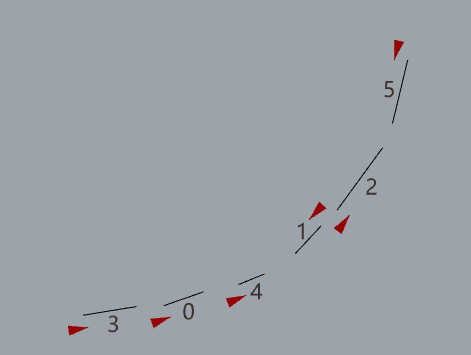As shown in the figure above, there are 6 lines, their order is not ordered according to the order, and their drawing directions are also different, their directions are some upwards, some downwards, so if you want to connect the curves directly, they must be chaotic. Looking at the result of the direct connection in the figure below, we can see that with the Grasshopper connection command, they are connected into a curve in a chaotic order.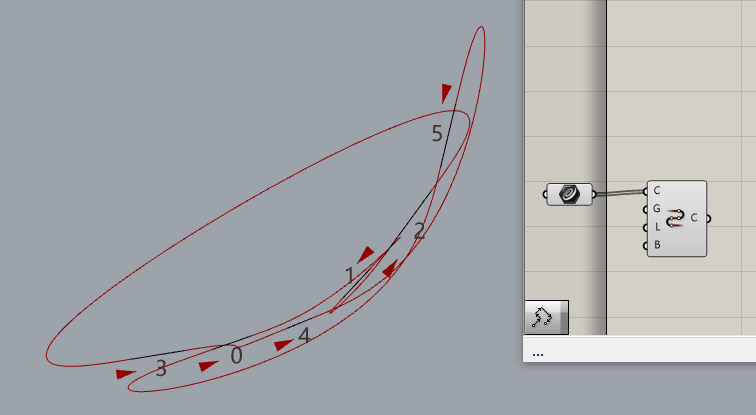If we want to get a regular curve, we must solve the following problems:

1. Sort the order of curves by rule

2. Uniform curve direction

Let’s solve the first problem first

Sort the order of curves by rule

To sort curves in Grasshopper, to put it plainly is to arrange points. Arrange points generally use the “Arrange Point Order by Curve” component. This operation can draw a curve through the point on the rhino, and then Grasshopper can get the curve and follow The distance from the point to the line is used to sort the point order.

The drawn curve, this curve does not need to be too precise.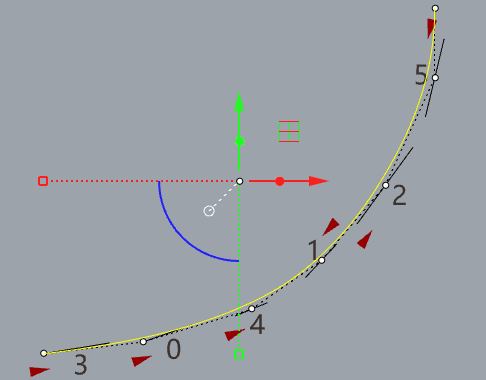Get the start point and end point of the line segment, and enter it into the “Sort Along Curve” component (Sort Along Curve)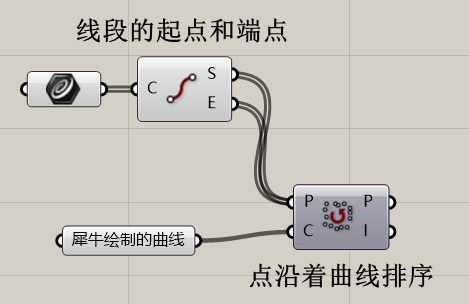Then the output points are divided into two groups of data, and the data of each group of two points are connected into a polyline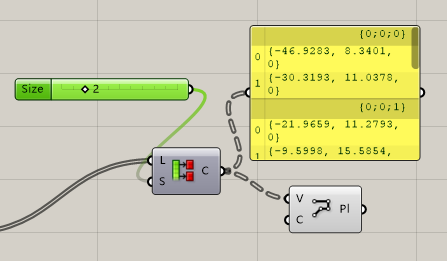At this time, the generated line segments should be arranged in order, and finally connect the data evenly.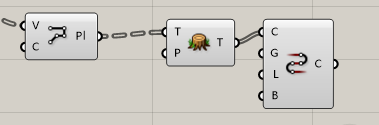Let’s take a look at the final result. We can see that the line segments are connected in sequence. In the result of the figure below, please pay attention to changing the parameter of [L port] to [FALSE], so that the curve is an open curve, not a closed curve.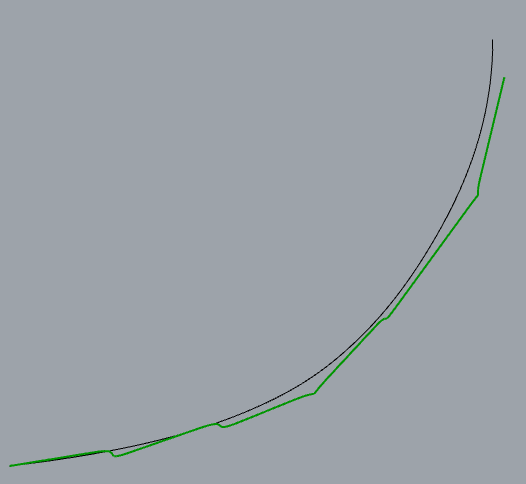Let’s take a look at the complete data logic. If you have any questions about Grasshopper, you can ask questions in the comment area below.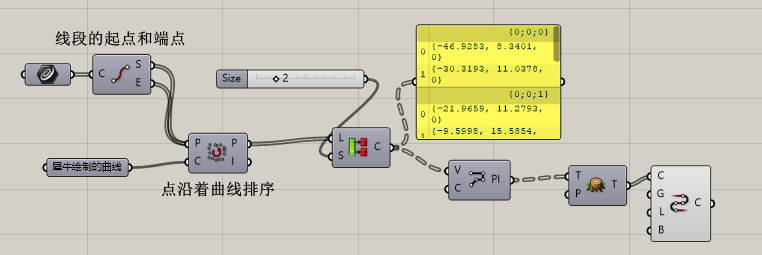Scroll to Top